Do you like the article?
Share it with others —
Use new possibilities of MetaTrader 5

#### Similar articles# Developing a trading Expert Advisor from scratch (Part 9): A conceptual leap (II)

MetaTrader 5Indicators | 27 June 2022, 16:214 7020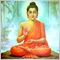### Introduction

In the previous part, we created a basic system which enables the use of templates within a floating window. Although we have made many changes, the code is not yet finished. This has been done intentionally in order to keep the explanation simple, because while the use of templates in floating windows is rather simple, the use of objects is something much more complicated. So, be ready for a completely new job.

In fact, the biggest difficulty related to the use of the objects which we utilize in order to create the CHART TRADE interface in the floating window is that MetaTrader 5 is not really intended for this purpose. Some readers may say that we could use the standard library for creating the CHART TRADE window. But I like to complicate things and I want to enable everyone to create their own interface the same way which we discussed a few articles ago. However, everything was simple in that article. Now we need to understand the restrictions in MetaTrader 5 in order to get around them.

### Planning

Let's start with the basics. The following code will behave as we expect:

```#property copyright "Daniel Jose"
#property indicator_chart_window
#property indicator_plots 0
//+------------------------------------------------------------------+
int OnInit()
{
long id = ChartID();
string sz0 = (string)ObjectsTotal(id, -1, -1) + (string)MathRand();
ObjectCreate(id, sz0, OBJ_CHART, 0, 0, 0);
ObjectSetInteger(id, sz0, OBJPROP_XDISTANCE, 10);
ObjectSetInteger(id, sz0, OBJPROP_YDISTANCE, 10);
ObjectSetInteger(id, sz0, OBJPROP_XSIZE, 300);
ObjectSetInteger(id, sz0, OBJPROP_YSIZE, 300);
ObjectSetInteger(id, sz0, OBJPROP_PRICE_SCALE, false);
ObjectSetInteger(id, sz0, OBJPROP_DATE_SCALE, false);

return INIT_SUCCEEDED;
}
//+------------------------------------------------------------------+
int OnCalculate(const int rates_total,
const int prev_calculated,
const int begin,
const double &price[])
{
return rates_total;
}
//+------------------------------------------------------------------+

```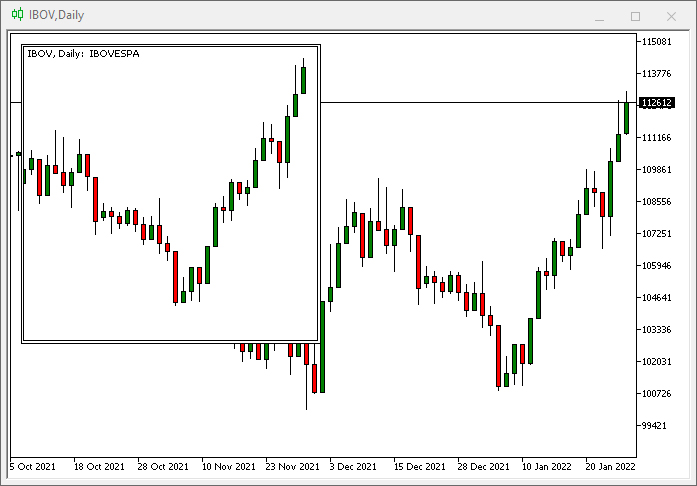There is nothing complicated here since the effect was exactly as expected. But MQL5 allows you to go a little further, although difficulties may arise when you try to do something beyond what the system was originally designed for. So, if we change the above code to something like the one below, things get interesting.

```int OnInit()
{
long id = ChartID(), handle;
string sz0 = (string)ObjectsTotal(id, -1, -1) + (string)MathRand(), sz1 = (string)MathRand();

ObjectCreate(id, sz0, OBJ_CHART, 0, 0, 0);
ObjectSetInteger(id, sz0, OBJPROP_XDISTANCE, 10);
ObjectSetInteger(id, sz0, OBJPROP_YDISTANCE, 10);
ObjectSetInteger(id, sz0, OBJPROP_XSIZE, 300);
ObjectSetInteger(id, sz0, OBJPROP_YSIZE, 300);
ObjectSetInteger(id, sz0, OBJPROP_PRICE_SCALE, false);
ObjectSetInteger(id, sz0, OBJPROP_DATE_SCALE, false);

handle = ObjectGetInteger(id, sz0, OBJPROP_CHART_ID);
ObjectCreate(handle, sz1, OBJ_CHART, 0, 0, 0);
ObjectSetInteger(handle, sz1, OBJPROP_XDISTANCE, 50);
ObjectSetInteger(handle, sz1, OBJPROP_YDISTANCE, 50);
ObjectSetInteger(handle, sz1, OBJPROP_XSIZE, 300);
ObjectSetInteger(handle, sz1, OBJPROP_YSIZE, 300);
ObjectSetInteger(handle, sz1, OBJPROP_PRICE_SCALE, false);
ObjectSetInteger(handle, sz1, OBJPROP_DATE_SCALE, false);
ChartRedraw(handle);

return INIT_SUCCEEDED;
}
```

We have added the highlighted lines to the code. If you run it on a chart, the result will be like this: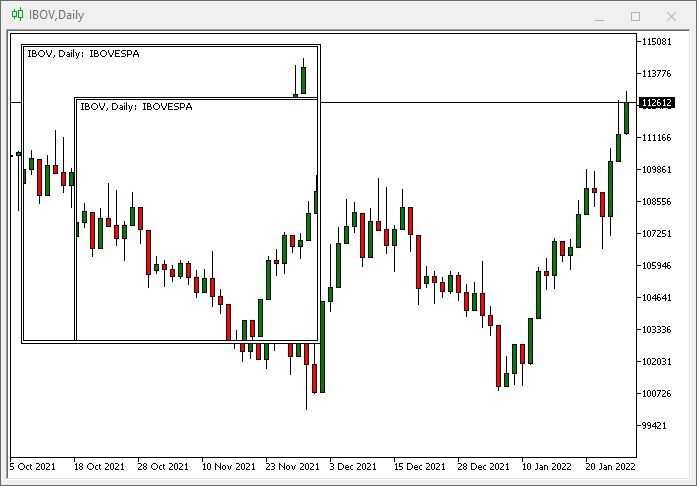What happened? We have placed a chart on a chart. We could place any object here, as MQL5 allows doing so, but this has both advantages and disadvantages. Take a look at the next change in the code in order to understand the advantage of this step.

```int OnInit()
{
long id = ChartID(), handle;
string sz0 = (string)ObjectsTotal(id, -1, -1) + (string)MathRand(), sz1 = (string)MathRand();

ObjectCreate(id, sz0, OBJ_CHART, 0, 0, 0);
ObjectSetInteger(id, sz0, OBJPROP_XDISTANCE, 10);
ObjectSetInteger(id, sz0, OBJPROP_YDISTANCE, 10);
ObjectSetInteger(id, sz0, OBJPROP_XSIZE, 300);
ObjectSetInteger(id, sz0, OBJPROP_YSIZE, 300);
ObjectSetInteger(id, sz0, OBJPROP_PRICE_SCALE, false);
ObjectSetInteger(id, sz0, OBJPROP_DATE_SCALE, false);
ObjectSetInteger(id, sz0, OBJPROP_SELECTABLE, true);
ObjectSetInteger(id, sz0, OBJPROP_SELECTED, true);

handle = ObjectGetInteger(id, sz0, OBJPROP_CHART_ID);
ObjectCreate(handle, sz1, OBJ_CHART, 0, 0, 0);
ObjectSetInteger(handle, sz1, OBJPROP_XDISTANCE, 50);
ObjectSetInteger(handle, sz1, OBJPROP_YDISTANCE, 50);
ObjectSetInteger(handle, sz1, OBJPROP_XSIZE, 300);
ObjectSetInteger(handle, sz1, OBJPROP_YSIZE, 300);
ObjectSetInteger(handle, sz1, OBJPROP_PRICE_SCALE, false);
ObjectSetInteger(handle, sz1, OBJPROP_DATE_SCALE, false);
ChartRedraw(handle);

return INIT_SUCCEEDED;
}

```

The addition of the highlighted lines has generated the following result: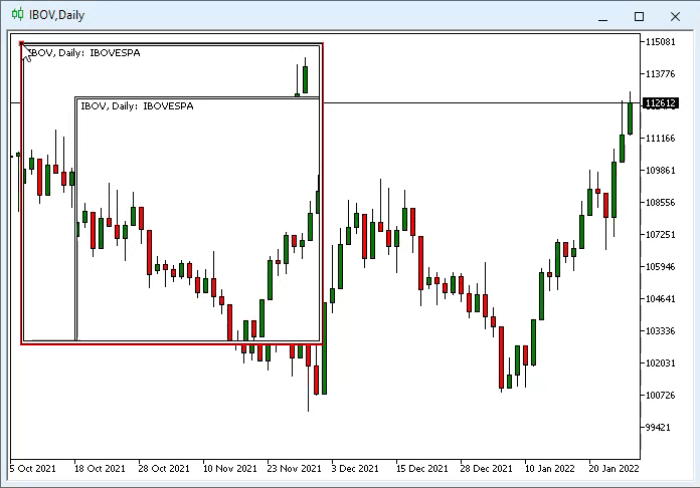This means that everything that is inside the object will remain inside the object. This is required when we use floating windows, as this greatly simplifies the control logic. But not everything is perfect: MetaTrader 5 was not originally designed for this. Therefore, a problem arises when one object is located inside another - we can't send events to internal objects. To understand this, let's implement a few more changes to the code. ow, the final code will be as follows:

```#property copyright "Daniel Jose"
#property indicator_chart_window
#property indicator_plots 0
//+------------------------------------------------------------------+
int OnInit()
{
long id = ChartID(), handle;
string sz0 = (string)ObjectsTotal(id, -1, -1) + (string)MathRand(), sz1 = (string)MathRand();

ObjectCreate(id, sz0, OBJ_CHART, 0, 0, 0);
ObjectSetInteger(id, sz0, OBJPROP_XDISTANCE, 10);
ObjectSetInteger(id, sz0, OBJPROP_YDISTANCE, 10);
ObjectSetInteger(id, sz0, OBJPROP_XSIZE, 300);
ObjectSetInteger(id, sz0, OBJPROP_YSIZE, 300);
ObjectSetInteger(id, sz0, OBJPROP_PRICE_SCALE, false);
ObjectSetInteger(id, sz0, OBJPROP_DATE_SCALE, false);
ObjectSetInteger(id, sz0, OBJPROP_SELECTABLE, true);
ObjectSetInteger(id, sz0, OBJPROP_SELECTED, true);

handle = ObjectGetInteger(id, sz0, OBJPROP_CHART_ID);
ObjectCreate(handle, sz1, OBJ_CHART, 0, 0, 0);
ObjectSetInteger(handle, sz1, OBJPROP_XDISTANCE, 50);
ObjectSetInteger(handle, sz1, OBJPROP_YDISTANCE, 50);
ObjectSetInteger(handle, sz1, OBJPROP_XSIZE, 300);
ObjectSetInteger(handle, sz1, OBJPROP_YSIZE, 300);
ObjectSetInteger(handle, sz1, OBJPROP_PRICE_SCALE, false);
ObjectSetInteger(handle, sz1, OBJPROP_DATE_SCALE, false);
ObjectSetInteger(handle, sz1, OBJPROP_SELECTABLE, true);
ObjectSetInteger(handle, sz1, OBJPROP_SELECTED, true);
ChartRedraw(handle);

return INIT_SUCCEEDED;
}
//+------------------------------------------------------------------+
int OnCalculate(const int rates_total,
const int prev_calculated,
const int begin,
const double &price[])
{
return rates_total;
}
//+------------------------------------------------------------------+
void OnChartEvent(const int id, const long &lparam, const double &dparam, const string &sparam)
{
if (id == CHARTEVENT_OBJECT_CLICK) Print(sparam);
}
//+------------------------------------------------------------------+

```

Here is the result of the code running in the platform: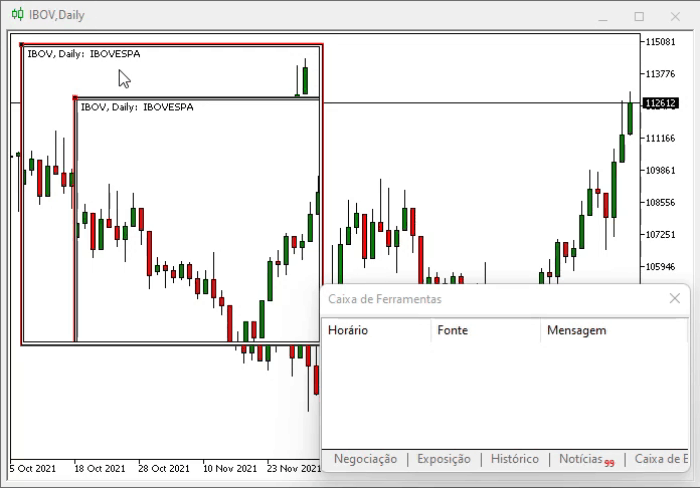Note that when we click on the inner object, a click is actually made on the outer object, and this is where things get complicated. But the programmer always strives to become an expert at solving problems: you have to solve problems in order to get the desired result. By using such knowledge, we will construct the system so as to create CHART TRADE in a floating window and to ensure that it is functional and has individual appearance.

There is one last phase in this planning process. Although this part is not so acute for modern computers, it still needs to be considered: optimization of processing time. The problem is connected with the number of operations which the processor has to perform, rather than the time it will take to process the information. The proposed floating window system contains four objects that should be able to move around reacting to your actions. Accordingly, any information placed in the viewport will be subject to the window's own modifications. At least CHART TRADE will increase the number of objects. And although there is no corresponding computational cost, the code becomes unpleasant and seems to be poorly optimized. We could simply add a control system and that would solve the problem. But there is a more elegant suggestion. Although it seems more time and effort consuming, it actually reduces the number of objects that need to be maintained and manipulated.

### Implementation

First, we will split the creation of the floating window into several steps to support code reuse. Next, we will create two new functions in the C_ChartFloating object class:

```//+------------------------------------------------------------------+
bool StageLocal01(string sz0, ENUM_TIMEFRAMES TimeFrame = PERIOD_CURRENT, int Scale = -1)
{
m_LimitX = (int)ChartGetInteger(Terminal.Get_ID(), CHART_WIDTH_IN_PIXELS);
m_LimitY = (int)ChartGetInteger(Terminal.Get_ID(), CHART_HEIGHT_IN_PIXELS);
if (m_MaxCounter >= def_MaxFloating) return false;
CreateBarTitle();
CreateCaption(sz0);
CreateBtnMaxMin();
CreateRegion(TimeFrame, Scale);
m_Win[m_MaxCounter].handle = ObjectGetInteger(Terminal.Get_ID(), m_Win[m_MaxCounter].szRegionChart, OBJPROP_CHART_ID);

return true;
}
//+------------------------------------------------------------------+
void StageLocal02(int x, int y, int w, int h)
{
y = (y < 0 ? m_MaxCounter * def_SizeBarCaption : y);
m_Win[m_MaxCounter].PosX        = -1;
m_Win[m_MaxCounter].PosY        = -1;
m_Win[m_MaxCounter].PosX_Minimized = m_Win[m_MaxCounter].PosX_Maximized = x;
m_Win[m_MaxCounter].PosY_Minimized = m_Win[m_MaxCounter].PosY_Maximized = y;
SetDimension(w, h, true, m_MaxCounter);
SetPosition(x, y, m_MaxCounter);
ChartRedraw(m_Win[m_MaxCounter].handle);
m_MaxCounter++;
}
//+------------------------------------------------------------------+

```

The new code adding a floating window will be as follows:

```bool AddIndicator(string sz0, int x = 0, int y = -1, int w = 300, int h = 200, ENUM_TIMEFRAMES TimeFrame = PERIOD_CURRENT, int Scale = -1)
{
if (!StageLocal01(sz0, TimeFrame, Scale)) return false;
ChartApplyTemplate(m_Win[m_MaxCounter].handle, sz0 + ".tpl");
m_Win[m_MaxCounter].szVLine = (string)ObjectsTotal(Terminal.Get_ID(), -1, -1) + (string)MathRand();
ObjectCreate(m_Win[m_MaxCounter].handle, m_Win[m_MaxCounter].szVLine, OBJ_VLINE, 0, 0, 0);
ObjectSetInteger(m_Win[m_MaxCounter].handle, m_Win[m_MaxCounter].szVLine, OBJPROP_COLOR, clrBlack);
StageLocal02(x, y, w, h);

return true;
}

```

This does not affect the already assembled system but provides its better use. Pay attention to the highlighted lines: now we will create a function to use our IDE. The beginning is shown below:

```bool Add_RAD_IDE(string sz0, int x, int y, int w, int h)
{
if (!StageLocal01(sz0, PERIOD_CURRENT, -1)) return false;
StageLocal02(x, y, w, h);
return true;
}

```

Pay attention that the highlighted lines are the same lines that we used in the previous code. I.e. we are reusing the code, and only the things that we need to adjust are different. Now we can inform our system that we have the tools to manage the IDE. We do this by modifying the C_TemplateChart object class. The code below shows exactly what will be changed in the function, so from now on we can focus on implementing a floating window with the IDE, since all the necessary support will already work correctly.

```void AddTemplate(void)
{

// .... Function code....

if (h == 0)
{
SetBase(m_Params.Param[TEMPLATE], (bIsSymbol ? m_Params.Param[TEMPLATE] : _Symbol), timeframe, i, w);
if (!ChartApplyTemplate(m_Info[m_Counter - 1].handle, m_Params.Param[TEMPLATE] + ".tpl")) if (bIsSymbol) ChartApplyTemplate(m_Info[m_Counter - 1].handle, "Default.tpl");
}
{
if ((h > 0) && (w > 0)) Add_RAD_IDE(m_Params.Param[TEMPLATE], 0, -1, w, h); else
{
C_Chart_IDE::Create(GetIdSubWinEA());
m_Info[m_Counter - 1].szVLine = "";
}
}else
{
if ((w > 0) && (h > 0)) AddIndicator(m_Params.Param[TEMPLATE], 0, -1, w, h, timeframe, i); else
{
m_Info[m_Counter - 1].szVLine = (string)ObjectsTotal(Terminal.Get_ID(), -1, -1) + (string)MathRand();
ObjectCreate(m_Info[m_Counter - 1].handle, m_Info[m_Counter - 1].szVLine, OBJ_VLINE, 0, 0, 0);
ObjectSetInteger(m_Info[m_Counter - 1].handle, m_Info[m_Counter - 1].szVLine, OBJPROP_COLOR, clrBlack);
}
}
}

```

Let's see how the code is configured to be as flexible as possible. This will prevent the system from turning into Frankenstein. As we modify the code, always keep this in mind - there is no need to rewrite the code from scratch and to check the same thing several times. Always try to check things only once. Then you can use and explore the things as much as possible before you have to do new tests. Thus, the system will grow with good premises while the code will remain sustainable and expandable over time.

If you run the system now, it will show something on the chart. But we need it to show at least the interface we have written earlier. Therefore, we need to make additional changes to the code. Now we have the following code:

```bool Add_RAD_IDE(string sz0, int x, int y, int w, int h)
{
if (!StageLocal01(sz0, PERIOD_CURRENT, -1)) return false;
StageLocal02(x, y, w, h);
return true;
}
```

Here is the app launch result: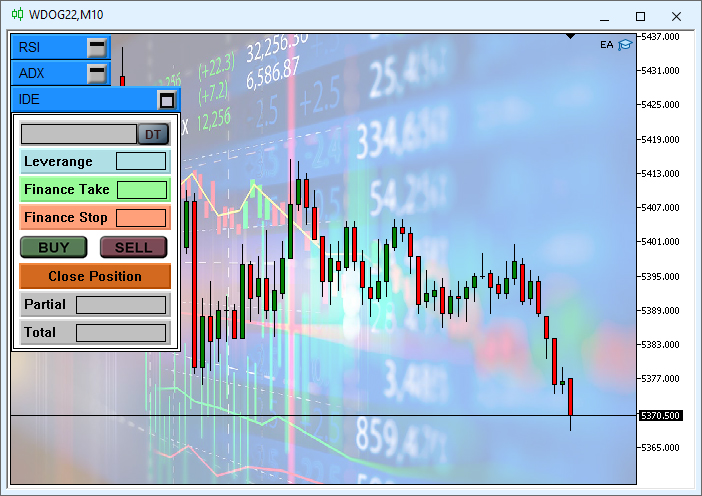It would be very good and beautiful if it were possible to access the objects that are in the template (the template is loaded in the line highlighted in the code above). However, it is not possible. And here is the important detail: instead of creating objects the way we considered earlier, we only create the objects that should be manipulated! This will save a lot of processing time when we have to move the window. We still have another problem but let us first solve the handling problem and make the system functional. Actually, this part is already done, it just needs some adjustment to make things work.

Let's start by making changes to the inheritance sequence between classes. We have to do this because we don't have multiple inheritance, so the new structure will be like this: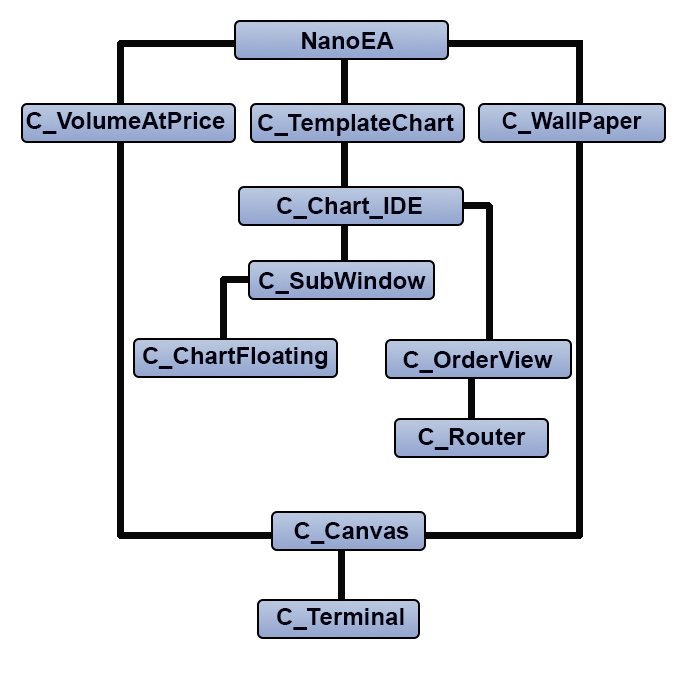But this change should not be a concern: a change in the inheritance sequence will not change the code at all but will allow it to be almost ready. The parts that have been changed are highlighted in the code below.

```bool Add_RAD_IDE(string sz0, int x, int y, int w, int h)
{
if ((w <= 0) || (h <= 0)) return false;
if (!StageLocal01(sz0, PERIOD_CURRENT, -1)) return false;
StageLocal02(x, y, w, h);
return true;
}
{
// ..... Código ....
if (h == 0)
{
SetBase(m_Params.Param[TEMPLATE], (bIsSymbol ? m_Params.Param[TEMPLATE] : _Symbol), timeframe, i, w);
if (!ChartApplyTemplate(m_Info[m_Counter - 1].handle, m_Params.Param[TEMPLATE] + ".tpl")) if (bIsSymbol) ChartApplyTemplate(m_Info[m_Counter - 1].handle, "Default.tpl");
}
{
m_Info[m_Counter - 1].szVLine = "";
}else
{
if ((w > 0) && (h > 0)) AddIndicator(m_Params.Param[TEMPLATE], 0, -1, w, h, timeframe, i); else
{
m_Info[m_Counter - 1].szVLine = (string)ObjectsTotal(Terminal.Get_ID(), -1, -1) + (string)MathRand();
ObjectCreate(m_Info[m_Counter - 1].handle, m_Info[m_Counter - 1].szVLine, OBJ_VLINE, 0, 0, 0);
ObjectSetInteger(m_Info[m_Counter - 1].handle, m_Info[m_Counter - 1].szVLine, OBJPROP_COLOR, clrBlack);
}
}
}
bool Create(bool bFloat)
{
m_CountObject = 0;
if ((m_fp = FileOpen("Chart Trade\\IDE.tpl", FILE_BIN | FILE_READ)) == INVALID_HANDLE) return false;

for (m_CountObject = eRESULT; m_CountObject <= eEDIT_STOP; m_CountObject++) m_ArrObject[m_CountObject].szName = "";
m_SubWindow = ((m_IsFloating = bFloat) ? 0 : GetIdSubWinEA());
m_szLine = "";
while (m_szLine != "</chart>")
{
if (m_szLine == "<object>")
{
if (m_szLine == "type")
{
if (m_szValue == "102") if (!LoopCreating(OBJ_LABEL)) return false;
if (m_szValue == "103") if (!LoopCreating(OBJ_BUTTON)) return false;
if (m_szValue == "106") if (!LoopCreating(OBJ_BITMAP_LABEL)) return false;
if (m_szValue == "107") if (!LoopCreating(OBJ_EDIT)) return false;
if (m_szValue == "110") if (!LoopCreating(OBJ_RECTANGLE_LABEL)) return false;
}
}
}
FileClose(m_fp);
DispatchMessage(CHARTEVENT_CHART_CHANGE, 0, 0, szMsgIDE[eLABEL_SYMBOL]);
return true;
}

bool LoopCreating(ENUM_OBJECT type)
{
#define macro_SetInteger(A, B) ObjectSetInteger(Terminal.Get_ID(), m_ArrObject[c0].szName, A, B)
#define macro_SetString(A, B) ObjectSetString(Terminal.Get_ID(), m_ArrObject[c0].szName, A, B)
int c0;
bool b0;
string sz0 = m_szValue;
while (m_szLine != "</object>") if (!FileReadLine()) return false; else
{
if (m_szLine == "name")
{
b0 = false;
StringToUpper(m_szValue);
for(c0 = eRESULT; (c0 <= eEDIT_STOP) && (!(b0 = (m_szValue == szMsgIDE[c0]))); c0++);
if (!b0 && m_IsFloating) return true; else c0 = (b0 ? c0 : m_CountObject);
m_ArrObject[c0].szName = StringFormat("%s%04s>%s", def_HeaderMSG, sz0, m_szValue);

//... The rest of the function...

}

```

This may seem strange. But there is nothing complicated here. Remember, when we do not use a floating window, the system is already capable of handling events running in our IDE. But because of that floating window, we have to rebuild everything. However, we do not have to do this from scratch — we will modify the existing code to add IDE in the right place. We only need to add the objects that receive events. These changes allow us to know whether we need to create all elements or only some of them.

After these changes, we have IDE information on the chart. But IDE objects will create a real mess since the objects are not associated with the floating window. Now this needs to be fixed.

First, we need to get the points where the floating window is located on the chart. It is enough to know the points of the object representing the window. But since stick to object-oriented programming, we'll need to make some changes, although the main result is the code below.

```//+------------------------------------------------------------------+
struct IDE_Struct
{
int     X,
Y,
Index;
bool    IsMaximized;
};
//+------------------------------------------------------------------+
class C_ChartFloating
{

// ... Class code ...

//+------------------------------------------------------------------+
void SetPosition(const int X, const int Y, const int c0)
{

// ... Function code ...

if (c0 == m_IDEStruct.Index)
{
m_IDEStruct.X = m_Win[c0].PosX + 3;
m_IDEStruct.Y = m_Win[c0].PosY + def_SizeBarCaption + 3;
m_IDEStruct.IsMaximized = m_Win[c0].IsMaximized;
}
}
//+------------------------------------------------------------------+

// ... Class code ...

//+------------------------------------------------------------------+
inline IDE_Struct GetIDE_Struct(void) const { return m_IDEStruct; }
//+------------------------------------------------------------------+
{
if ((w <= 0) || (h <= 0)) return false;
if (!StageLocal01(sz0, PERIOD_CURRENT, -1)) return false;
m_IDEStruct.Index = m_MaxCounter;
StageLocal02(x, y, w, h);
return true;
}
//+------------------------------------------------------------------+

//... The rest of the class code
}
```

Since the solution focuses on one window — our CHART TRADER — there is no point in making things more complicated after this point. Now we can correctly position objects in the window, at least initially. This is done in the following function.

```void Resize(int x)
{
for (int c0 = 0; c0 < m_CountObject; c0++) if (m_IsFloating)
{
ObjectSetInteger(Terminal.Get_ID(), m_ArrObject[c0].szName, OBJPROP_XDISTANCE, GetIDE_Struct().X + m_ArrObject[c0].iPosX);
ObjectSetInteger(Terminal.Get_ID(), m_ArrObject[c0].szName, OBJPROP_YDISTANCE, GetIDE_Struct().Y + m_ArrObject[c0].iPosY);
}else   ObjectSetInteger(Terminal.Get_ID(), m_ArrObject[c0].szName, OBJPROP_XDISTANCE, x + m_ArrObject[c0].iPosX);
};
```

The above function provides the correct initial positioning of the objects, and the result can be seen below: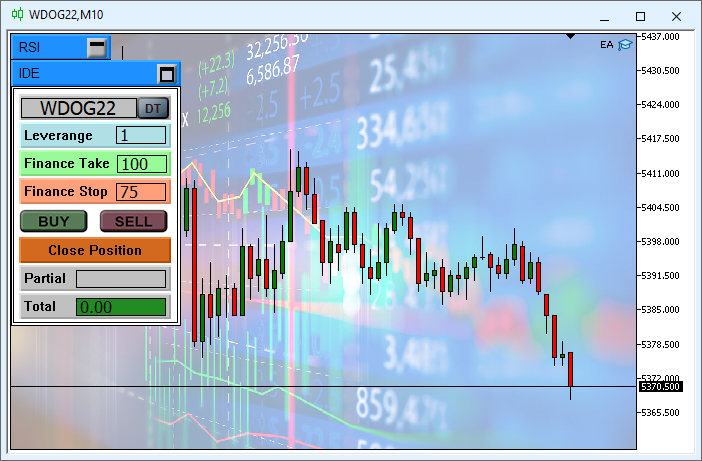In addition to the correct initial positioning, objects already react to events. However, the system still has bugs, which need to be fixed. Here is the first thing we are going to change: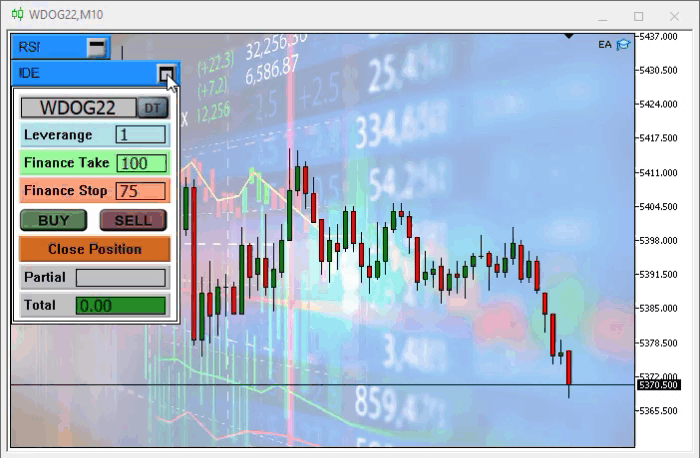As you can see, the window has been minimized but the objects remain on the screen. This is because objects are not inside the window area ( I explained earlier why). This needs to be fixed before we move on. It can be done by a simple code modification shown below:

```void Resize(int x)
{
for (int c0 = 0; c0 < m_CountObject; c0++) if (m_IsFloating)
{
ObjectSetInteger(Terminal.Get_ID(), m_ArrObject[c0].szName, OBJPROP_XDISTANCE, GetIDE_Struct().X + m_ArrObject[c0].iPosX + (GetIDE_Struct().IsMaximized ? 0 : Terminal.GetWidth()));
ObjectSetInteger(Terminal.Get_ID(), m_ArrObject[c0].szName, OBJPROP_YDISTANCE, GetIDE_Struct().Y + m_ArrObject[c0].iPosY + (GetIDE_Struct().IsMaximized ? 0 : Terminal.GetHeight()));
}else   ObjectSetInteger(Terminal.Get_ID(), m_ArrObject[c0].szName, OBJPROP_XDISTANCE, x + m_ArrObject[c0].iPosX);
};
```

The result is as follows: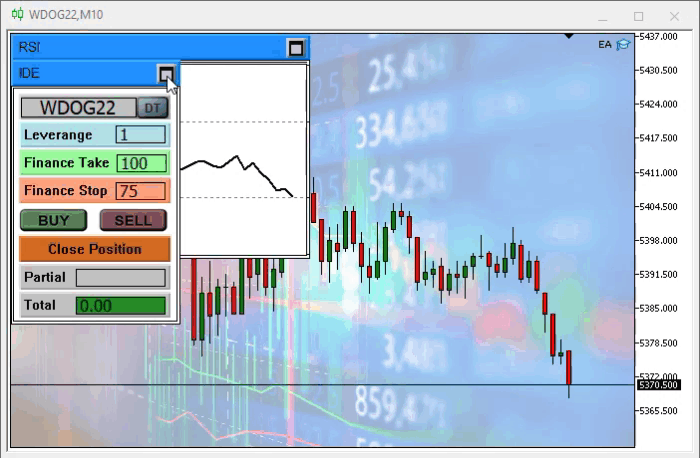Now let's fix the issue with the movement. It can be solved using the below code

```void DispatchMessage(int id, long lparam, double dparam, string sparam)
{
static double AccumulatedRoof = 0.0;
bool            b0;
double  d0;
static int px = -1, py = -1;

C_ChartFloating::DispatchMessage(id, lparam, dparam, sparam);
if (m_CountObject < eEDIT_STOP) return;
switch (id)
{
case CHARTEVENT_MOUSE_MOVE:
if ((GetIDE_Struct().X != px) || (GetIDE_Struct().Y != py))
{
px = GetIDE_Struct().X;
py = GetIDE_Struct().Y;
Resize(-1);
}
break;

//... The rest of the function ...
```

The final result is as follows: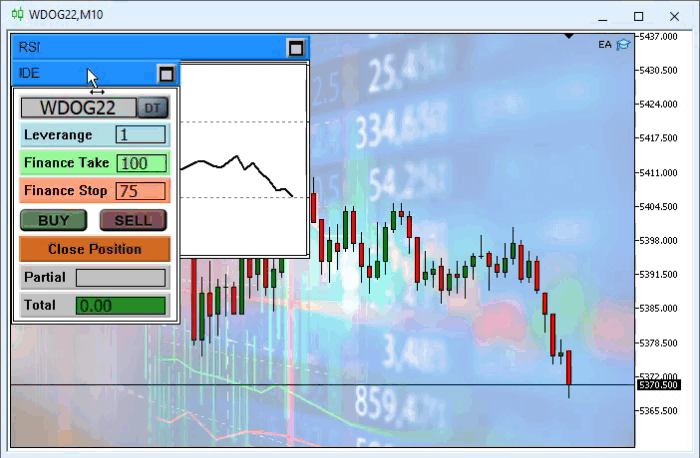### Conclusion

See how great programming is: we start with a problem, and then, without major changes to the code, we solve problems one by one, and in the end we have working code with the smallest amount of code possible.

Important! If you see strange information on the Chart Trade background, it is due to update 3228, which makes objects with color in clrNONE opaque. Use the attached IDE file which fixes the issue.

Translated from Portuguese by MetaQuotes Ltd.
Original article: https://www.mql5.com/pt/articles/10363

Attached files |
EA_1.09.zip (3617.03 KB)
IDE.tpl (10.13 KB)

#### Other articles by this author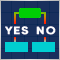Data Science and Machine Learning (Part 05): Decision Trees
Decision trees imitate the way humans think to classify data. Let's see how to build trees and use them to classify and predict some data. The main goal of the decision trees algorithm is to separate the data with impurity and into pure or close to nodes.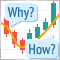Learn how to design a trading system by Ichimoku
Here is a new article in our series about how to design a trading system b the most popular indicators, we will talk about the Ichimoku indicator in detail and how to design a trading system by this indicator.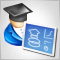Developing a trading Expert Advisor from scratch (Part 10): Accessing custom indicators
How to access custom indicators directly in an Expert Advisor? A trading EA can be truly useful only if it can use custom indicators; otherwise, it is just a set of codes and instructions.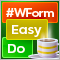DoEasy. Controls (Part 4): Panel control, Padding and Dock parameters
In this article, I will implement handling Padding (internal indents/margin on all sides of an element) and Dock parameters (the way an object is located inside its container).